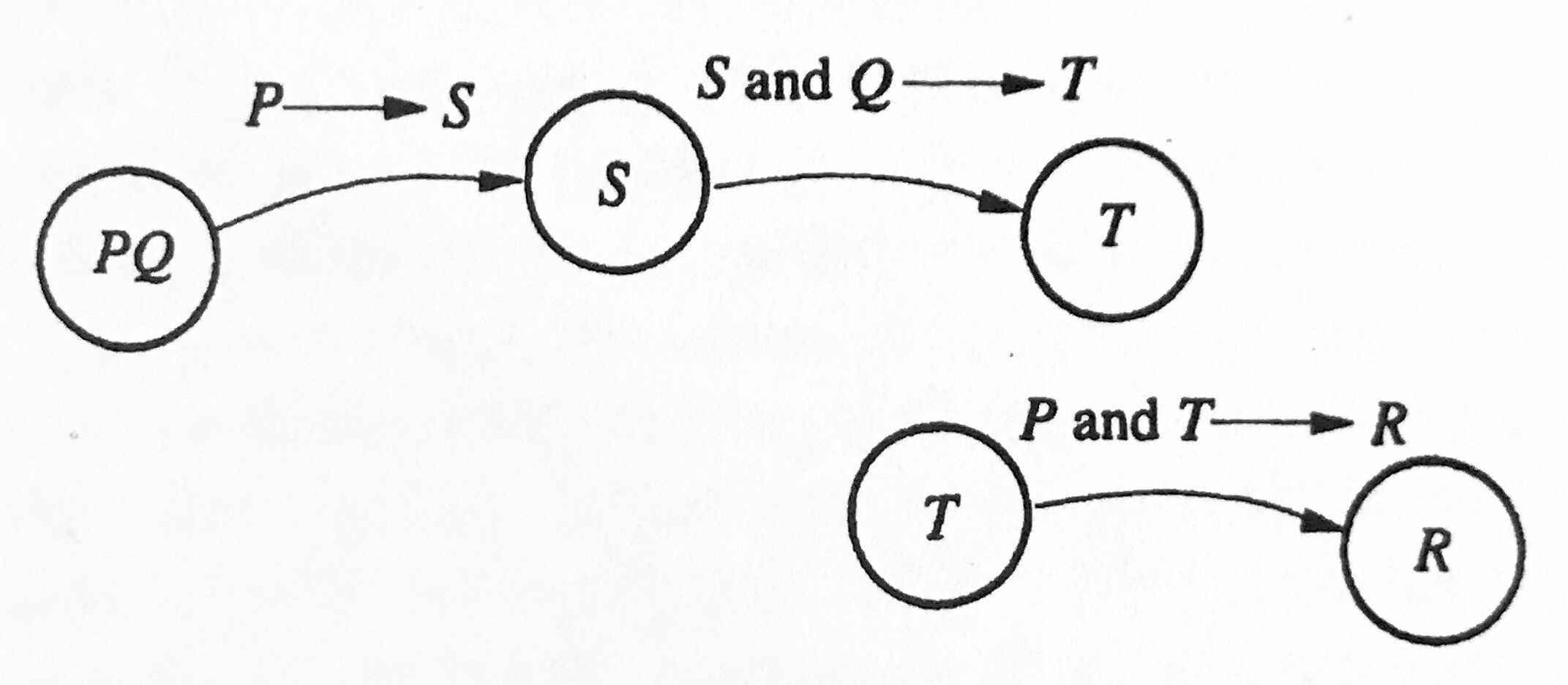# How to discover proofs?

### 如何寻找一个“证明”？

• 首先，我们可以先写下一个列表，_列出你所已知的东西_（比如：前提假设、关键对象的定义等），同时，_列出你需要证明的东西_（也就是结论，有些时候可以从定义去复述结论）；
• 然后，搜索可能将二者联系起来的那些定理。如果已知假设中，包含了你所知道的某一定理的假设条件，那么我们就很可能相应的推导出这一定理的结论——这是正向的方式；或者，你发现某一定理的结论和待证结论相同，那么该定理所需要满足的假设也就是你接下来要针对的新目标了——这是反向的方式。强烈建议同时利用正向、反向两种思维来寻求证明。### 如何书写证明？

**证明**： 我们要证明 $R$ 成立。但根据某某定理有 $P$ 和 $T$ 推出 $R$；而我们已知 $P$，只需证明 $T$ 成立就完成了证明。但我们注意到，根据某某定义 知 $P$ 能推出 $S$，同时根据前面的某某引理， $S$ 和 $Q$ 能推出 $T$。 综上，定理证明完毕。QED

**证明**： 假设 $P$ 与 $Q$成立。由某某定义，$P$ 推出 $S$，同时由前面的某某引理， $S$ 和 $Q$ 推出 $T$。于是我们同时有 $P$ 和 $T$ 成立。依据某某定理之假设，我们得到结论 $R$ 。QED

Zengfk

2019-06-27

2019-06-28